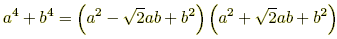# factoring rules

Factoring Rules

Algebra formulas for factoring.

 1.   x2 – (r + s)x + rs = (x – r)(x – s) 2.   x2 + 2ax + a2 = (x + a)2  and  x2– 2ax + a2 = (x – a)2 3.   Difference of squares:  a2– b2 = (a – b)(a + b) 4.   Difference of cubes:  a3– b3 = (a – b)(a2 + ab + b2) 5.   a4– b4 = (a – b)(a3 + a2b + ab2 + b3) = (a – b) [ a2(a + b) + b2(a + b) ] = (a – b)(a + b)(a2 + b2) or, more simply,   a4– b4 = (a2 – b2)(a2 + b2) = (a – b)(a + b)(a2 + b2) 6.   a5– b5 = (a – b)(a4 + a3b + a2b2 + ab3 + b4) 7.   an– bn = (a – b)(an– 1 + an– 2b + an– 3b2 + ··· + abn– 2 + bn– 1) 8.   Sum of cubes:  a3+ b3 = (a + b)(a2 – ab + b2) 9.   a5+ b5 = (a + b)(a4 – a3b + a2b2 – ab3 + b4) 10.   a7+ b7 = (a + b)(a6 – a5b + a4b2 – a3b3 + a2b4 – ab5 + b6) 11.   If n is odd, then  an+ bn = (a + b)(an– 1 – an– 2b + an– 3b2 – ··· + a2bn– 3 – abn– 2 + bn– 1) 12.   Sum of squares:  a2+ b2 = (a – bi)(a + bi)      Note:  a2+ b2  does not factor using real numbers. 13.